# AP Statistics : How to find the percentiles for a data set

## Example Questions

### Example Question #1 : How To Find The Percentiles For A Data Set

The 70th and 80th percentiles of a set of scores are 78 and 86, respectively; the interquartile range of the scores is 41. Which of these scores is more likely than the others to be at the 25th percentile?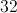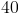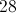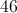Explanation:

The interquartile range of a set of scores is the difference between the third and first quartile - that is, the difference between the 75th and 25th percentiles. The 75th percentile is between 78 and 86, so, if 41 is subtracted from those numbers, the upper and lower bounds of the 25th percentile can be found.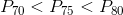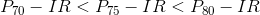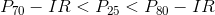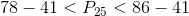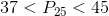Of our choices, only 40 falls in this range.

### Example Question #2 : How To Find The Percentiles For A Data Set

The following data set represents Mr. Marigold's students' scores on the final. If you got a 90 on the final, what percentile does that place you in?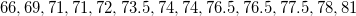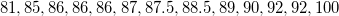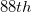percentile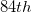percentile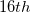percentile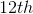percentilepercentile

Explanation:

Your percentile is the percent of the data set that is at your score or below.

There are 26 students in this particular class.

Of those students, 23 students were at a 90 or below.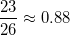, or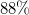.

### Example Question #3 : How To Find The Percentiles For A Data Set

The following data set represents Mr. Marigold's students' scores on the final. If you are in the 46th percentile, what did you score?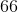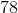Explanation:

If you scored in the 46th percentile, that means that 46% of your classmates scored the same as or worse than you.

This class has 26 students, and 46% of 26 is about 12.

Starting at 66 and counting 12 students forward would give you a 78.

This means that if you scored a 78, 12 students either had the same score or worse, so that is the 46th percentile.

### Example Question #4 : How To Find The Percentiles For A Data Set

The following data set represents Mr. Marigold's students' scores on the final. If you are in the 96th percentile, what did you score?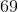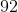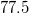Explanation:

If you scored in the 96th percentile, that means that 96% of your classmates scored the same as or worse than you.

This class has 26 students, and 96% of 26 is about 25.

If 25 of your classmates did the same as or worse than you, that means only one person did better than you - nice work.

You must have gotten a 92, because it's the second-best score.

### Example Question #5 : How To Find The Percentiles For A Data Set

The following data set represents Mr. Marigold's students' scores on the final. If you got a 74 on the final, what percentile does that place you in?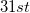percentile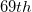percentile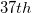percentile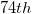percentilepercentile

Explanation:

If you scored a 74, you can count and discover that eight students did worse than or the same as you.

There are a total of 26 students in the class, so 8 students would be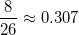or around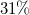.

This places you in the 31st percentile.

### Example Question #6 : How To Find The Percentiles For A Data Set

Your class' scores on a recent quiz was listed on the board.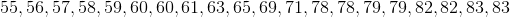Calculate the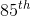percentile.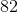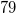Explanation:

To find the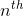percentile, we find the product of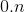and the number of itemsin the set.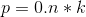We then round that numberup if it is not a whole number, and the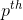term in the set is thepercentile.

For this problem, to find thepercentile, we find that there are 20 items in the set. We find the product to be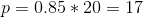Since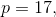in order to find thepercentile we find the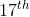term in the set, which is### Example Question #7 : How To Find The Percentiles For A Data Set

Your gym class' shot put distances are listed in the set below.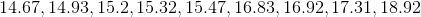Which score is the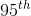percentile?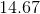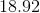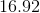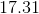Explanation:

To find thepercentile, we find the product ofand the number of itemsin the set.We then round that numberup if it is not a whole number, and theterm in the set is thepercentile.

For this problem, to find thepercentile, we find that there areitems in the set. We find the product to be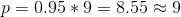Since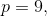in order to find thepercentile we find the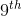term in the set, which is